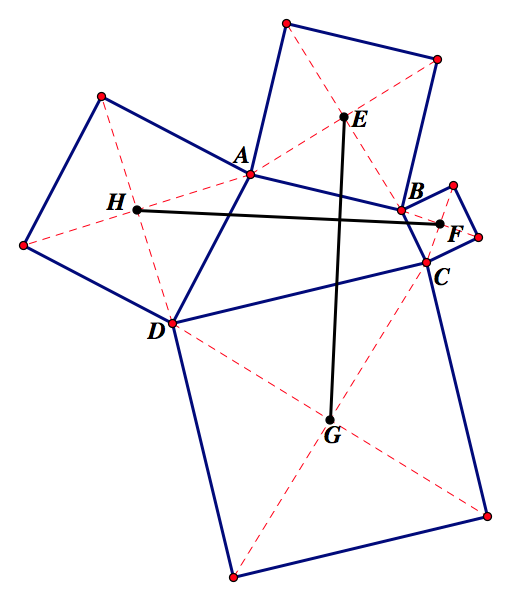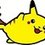# Proofs using Complex NumbersHey, everyone! For those of you who don't know me (most of you), I'm Sotiri and I'm a freshman at the University of Chicago. I am a member of the TorqueGroup (aka Torque Team) hashtag and hope to bring everyone some interesting or thought-provoking topics in the near future from my studies in math both in and out of school :)

With that in mind, I think a good topic for my first post is "proofs using complex numbers." Knowing how to do said proofs is very important, as very often complex numbers can be used to greatly simplify problems involving other topics, especially geometry and trigonometry. We should all take a moment to thank Euler for his marvelous discovery of the formula $e^{i\theta} = \cos(\theta) + i\sin(\theta)$. It truly is amazing.

Anyways, I'll demonstrate how to use the concept of complex numbers to prove one of the most famous theorems in all of mathematics: Heron's Formula! I originally found this proof in this paper on Art of Problem Solving, but I do not know who originally came up with it. Regardless, it is one of my favorite proofs, and I will reconstruct it here with plentiful explanation.https://i.imgur.com/80rHPKr.png

In the image above, $I$ is the incenter of $\bigtriangleup ABC$. This provides justification as to why the three segments emanating from $I$ and perpendicular to the sides are congruent and have length $r$ (they are radii of the incircle of $\bigtriangleup ABC$). Also, we can see that, as labeled, the central angles come in congruent pairs, since the incenter is the intersection of the angle bisectors of the triangle. Finally, since tangents from a point to a given circle are congruent, we see that the sub-segments on the sides of the triangle also come in congruent pairs.

We let $b = y + z, c = x + z, d = x + y$. Clearly, the semiperimeter $s = x + y + z$ and $\alpha + \beta + \gamma = \pi$. Now we introduce complex numbers into the problem by setting $r + ix = ue^{i\alpha}, r + iy = ve^{i\beta}, r + iz = we^{i\gamma}$. This is just a simple application of Euler's formula after defining a proper set of coordinates. For example:https://i.imgur.com/tiysGNn.png

We multiply everything together to get

$\begin{array}{rcl} (r + ix)(r + iy)(r + iz) & = & (ue^{i\alpha})(ve^{i\beta})(we^{i\gamma}) \\ & = & uvwe^{i(\alpha + \beta + \gamma)} \\ & = & uvwe^{i\pi} \\ & = & -uvw \end{array}$

so that the imaginary part of the product is $0$. However, we note that $(r + ix)(r + iy)(r + iz) = r^3 + r^2(x + y + z)i - r(xy + yz + zx) - xyzi$ and so $r^2(x + y + z) - xyz = 0$. It follows that $r = \sqrt{\frac{xyz}{x + y + z}}$.

We remind ourselves that $xyz = (s - b)(s - c)(s - d)$ and $x + y + z = s$, and so $r = \sqrt{\frac{(s - b)(s - c)(s - d)}{s}}$. But we know that we can express the area as $A = rs$ with the exact same $r$ and $s$ as we've already been using (it is well-known that the area is the product of the inradius and the semiperimeter), and so we finally get $A = rs = \sqrt{s(s - b)(s - c)(s - d)}$, as desired. $\square$

I hope the proof itself was clear, interesting, and shed some light on how powerful complex numbers can be in geometric proofs. It should be noted that we did almost no algebra at all other than the simple expansion of the product. The problem took a bit of time to set up, but after that, everything was very straightforward.

If you guys are interested in seeing more, I'd be happy to work through more complex-number proofs, especially of old IMO problems. Otherwise, please do let me know if there are other topics I should write about. For now, you all should try to solve this problem using complex numbers:https://i.imgur.com/WYlVOaa.png

The above figure shows an arbitrary quadrilateral with a square drawn on each of its sides. Show that $\overline{EG} \cong \overline{FH}$ and that the two segments are perpendicular.Note by Sotiri Komissopoulos
7 years, 5 months ago

This discussion board is a place to discuss our Daily Challenges and the math and science related to those challenges. Explanations are more than just a solution — they should explain the steps and thinking strategies that you used to obtain the solution. Comments should further the discussion of math and science.

When posting on Brilliant:

• Use the emojis to react to an explanation, whether you're congratulating a job well done , or just really confused .
• Ask specific questions about the challenge or the steps in somebody's explanation. Well-posed questions can add a lot to the discussion, but posting "I don't understand!" doesn't help anyone.
• Try to contribute something new to the discussion, whether it is an extension, generalization or other idea related to the challenge.
• Stay on topic — we're all here to learn more about math and science, not to hear about your favorite get-rich-quick scheme or current world events.

MarkdownAppears as
*italics* or _italics_ italics
**bold** or __bold__ bold
- bulleted- list
• bulleted
• list
1. numbered2. list
1. numbered
2. list
Note: you must add a full line of space before and after lists for them to show up correctly
paragraph 1paragraph 2

paragraph 1

paragraph 2

[example link](https://brilliant.org)example link
> This is a quote
This is a quote
    # I indented these lines
# 4 spaces, and now they show
# up as a code block.

print "hello world"
# I indented these lines
# 4 spaces, and now they show
# up as a code block.

print "hello world"
MathAppears as
Remember to wrap math in $$ ... $$ or $ ... $ to ensure proper formatting.
2 \times 3 $2 \times 3$
2^{34} $2^{34}$
a_{i-1} $a_{i-1}$
\frac{2}{3} $\frac{2}{3}$
\sqrt{2} $\sqrt{2}$
\sum_{i=1}^3 $\sum_{i=1}^3$
\sin \theta $\sin \theta$
\boxed{123} $\boxed{123}$

## Comments

Sort by:

Top Newest

Thanks for reading! I hope you enjoyed the post. Feel free to offer suggestions for next time and post your solutions to the second problem! :)

- 7 years, 5 months ago

Log in to reply

The proof of Heron's formula using complex numbers was published in American Mathematical Monthly according to:

http://www.artofproblemsolving.com/blog/363

- 7 years, 5 months ago

Log in to reply

Suppose that the four vertices $A,B,C,D$ are represented by complex numbers $a,b,c,d$. Now, remark that the square that has $AB$ as its side has vertices $a, a- i(b-a), b-i(b-a), b$ so we can calculate its center as:

$\frac{a+[a-i(b-a)]+[b-i(b-a)]+b}{4} = \frac{a+b+i(a-b)}{2}$.

Analogously calculate the centers of the other squares. So now, the line segment from the center of the square calculated above to the opposite one can be rewritten as,

$\beta = \frac{(c+d-a-b) + i(c-d-a+b) }{2}$

Similarly,

$\gamma = \frac{(d+a-b-c) + i(d-a-b+c)}{2}$

Now, just notice that $\gamma = i \beta$. The conclusion is immediate. ■

This post is just awesome :) Good job Sotiri! Personally, I would wish to see how to apply complex numbers into olympiad-style geometry problems since complex numbers are nothing but a mystery to me. I hope this isn't too much to ask and thank you in advance.

- 7 years, 5 months ago

Log in to reply

Checkout #26 of Fall 2013 OMO for an Olympiad level problem using complex numbers to solve:

http://internetolympiad.org/archive/OMOFall13/OMOFall13Probs.pdf

- 7 years, 5 months ago

Log in to reply

Thanks so much for the post. I agree with Anqi, I only know about euler's formula and roots of unity, but I would like to see how they can be applied to olympiad geometry problems. It would be great if you post a problem that isn't suited to complex numbers and a problem that is. :)

- 7 years, 5 months ago

Log in to reply

One of my other favourites is the trig identities with complex numbers. :-0

Let's say we have the following:

$cos(x+y)+isin(x+y)={ e }^{ i(x+y) }$ (Pretty much Euler's Identity).

Using exponent properties,

$cos(x+y)+isin(x+y)={ e }^{ ix }{ e }^{ iy }$

Using Euler's Identity. That's the same as: $(cos(x)+isin(x))(cos(y)+isin(y))$

$\Rightarrow$ Expanding it gives us: $(cos(x)cos(y) - sin(x)sin(y)) + i(cos(x)sin(y) + sin(x)cos(y))$

The real part: $(cos(x)cos(y) - sin(x)sin(y)) = cos(x+y)$ From Euler's Identity.

The imaginary part: $cos(x)sin(y) + sin(x)cos(y) = sin(x+y)$

Because, ${e}^{ix} = cos(x) + isin(x)$, the real part is the cosine and the imaginary part is the sine. :'-)

$Q.E.D$ I thought that was quite fun. :-0

Log in to reply

Woha amazing

- 7 years ago

Log in to reply

Thank you so much for this! The post was simply amazing!

I'll be looking forward to more posts like this [not necessarily on this topic]. I'm now following you!

- 7 years, 5 months ago

Log in to reply

You go to uchicago? Hopefully I'll see you there :P applying there for next fall as an undergraduate :)

- 7 years, 5 months ago

Log in to reply

I do :) Good luck to you next year! Even though they usually don't accept 24-year-olds :O.;O;O;O Heh :P If you have any questions about the school, feel free to ask me!

- 7 years, 5 months ago

Log in to reply

I'm applying this year (within the week, to be exact), with hopes of joining in the fall :P

What did you write your essay on? Uchicago is notorious for their, eh, quirky essay prompts, and I'm planning on thinking on writing it about a joke. Not really asking this for advice, I'm just curious

- 7 years, 5 months ago

Log in to reply

Oh, I misunderstood what you meant ^^ Sorry. I see.

They have that one "Explain your favorite joke" prompt this year, right? That sounds like something that would be fun to write about lol. I wrote mine using the "Past for a present" prompt they had last year. You had to come up with a background story for a present you'd received, and I wrote mine about a stuffed toy bird :P

- 7 years, 5 months ago

Log in to reply

Yeah, seriously, how is it that you're 23 and you're in high school?

- 7 years, 4 months ago

Log in to reply

He's not actually 23.

- 7 years, 4 months ago

Log in to reply

Phew...

- 7 years, 4 months ago

Log in to reply

Ha ha !!

- 7 years, 3 months ago

Log in to reply

He had me going.

- 7 years ago

Log in to reply

This is an amazing article and was a really good read! Good job Sotiri!

- 7 years, 5 months ago

Log in to reply

It was Mr. Miles Edwards

- 7 years ago

Log in to reply

The Latex portion is not visible so can not comment on your interesting wright up. Probably after some time it may be OK.

- 6 years, 8 months ago

Log in to reply

Great proof, thanks! It's nice to see complex numbers in action and it certainly shows how useful they can be :) . One topic of interest that popped to mind is the proof that R $\geq$2r where R = radius of circumcircle and r = radius of incircle (Euler's Theorem in geometry) - I've never been able to find an accessible/complete proof (the one on wikipedia needs a clearer explanation).

- 5 years, 11 months ago

Log in to reply

×

Problem Loading...

Note Loading...

Set Loading...